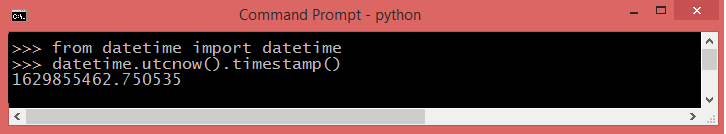Python Tutorial

# Get Current time | Python

In Python, the datetime module provides functions to work with dates and times. To obtain the current date and time, you can use the datetime class along with the datetime.now() method. Here's a detailed explanation with examples:

## Getting Current Date and Time

from datetime import datetime current_datetime = datetime.now() print("Current date and time:", current_datetime) # Output: Current date and time: 2023-08-09 15:30:00.123456

In this example, the datetime.now() method retrieves the current date and time, including microseconds. The result is stored in the current_datetime variable and displayed.

## Get current time - python3**datetime.utcnow() is a non-timezone aware object.

## Formatting Date and Time

formatted_datetime = current_datetime.strftime("%Y-%m-%d %H:%M:%S") print("Formatted date and time:", formatted_datetime) # Output: Formatted date and time: 2023-08-09 15:30:00

You can use the strftime() method to format the date and time. In this case, %Y represents the year, %m the month, %d the day, %H the hour, %M the minute, and %S the second.

## Date and Time Components

year = current_datetime.year month = current_datetime.month day = current_datetime.day hour = current_datetime.hour minute = current_datetime.minute second = current_datetime.second
print("Year:", year) print("Month:", month) print("Day:", day) print("Hour:", hour) print("Minute:", minute) print("Second:", second)

You can extract individual components of the date and time using attributes like year, month, day, hour, minute, and second.

## Date and Time Arithmetic

from datetime import timedelta one_week_later = current_datetime + timedelta(weeks=1) print("One week later:", one_week_later) # Output: One week later: 2023-08-16 15:30:00.123456

Using the timedelta class from the datetime module, you can perform date and time arithmetic. In this example, timedelta(weeks=1) adds one week to the current date and time.

### Conclusion

The datetime module in Python offers extensive functionalities for working with dates and times, making it convenient to obtain, format, manipulate, and compute different aspects of temporal data.